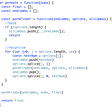# Recursive Algorithms

## How Recursion Works in Memory ?

1. Stack
2. Static data area
3. Code area
4. Heap
1. Incoming parameters
2. Return value
3. Temporary storage
4. Saved state information
5. Outgoing parameters

# Base cases and Recursion

## Basic Recursion

`sum(5)5 + sum(4)5 + (4 + sum(3))5 + (4 + (3 + sum(2)))5 + (4 + (3 + (2 + sum(1))))12 + 1; // unwinding start3 + 3;4 + 6;5 + 10;15`

## Tail Recursion

`sum(5)5 + sum(4)5 + (4 + sum(3))5 + (4 + (3 + sum(2)))5 + (4 + (3 + (2 + sum(1))))5 + (4 + (3 + (2 + 1)))15`
• If exponent is 0, then power is 1. This is the base condition of our recursive function.
• If exponent is negative, then power is `1 / (x ^ -y)`. Which uses recursive call to `_pow_recursion`function for computing the value of `(x ^ -1)` i.e. `1 / _pow_recursion(base, -expo)`.
• If exponent is positive, then calculate power normally using `x ^ y`. Computing `x ^ y` also makes a recursive call i.e. `base * _pow_recursion(base, expo - 1)`

# Using Divide and Conquer.

--

--

--

## More from Cristian Mendoza

Developer | Software engineer in process | Facebook | instagram | Student in Holberton school

Love podcasts or audiobooks? Learn on the go with our new app.

## TIME IS RUNNING OUT. PROTECT YOUR VMWARE INVESTMENTS## Introducing the Cloud Native Compute Foundation (CNCF)## Essential Data Structures and Algorithms for Coding Interviews## TOP 10 PROGRAMMING LANGUAGES USED IN AI CHATBOT BUILDING## Protected view-only Postgres schemas## Cristian Mendoza

Developer | Software engineer in process | Facebook | instagram | Student in Holberton school

## Algorithms: Recursion/BackTracking, Permutations## Arrays for the uninitiated